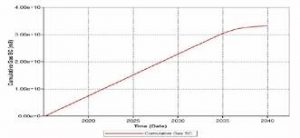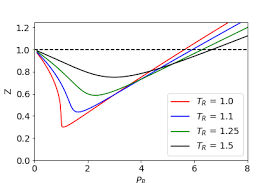## How to Calculate and Solve for Gas Recovery Factor (Production) | Reservoir Fluid FlowThe image above represents a gas recovery factor (G).

To compute for a gas recovery factor (production), two essential parameters are needed and these parameters are cumulative gas production (GP) and initial gas in place (G).

The formula for calculating the gas recovery factor (G):

RFG = Gp / G

Where:

RFG = Gas Recovery Factor (G)
GP = Cumulative Gas Production
G = Initial Gas in Place

Let’s solve an example;
Find the gas recovery factor (G) when the cumulative gas production is 13 and the initial gas in place is 17.

This implies that;

GP = Cumulative Gas Production = 13
G = Initial Gas in Place = 17

RFG = Gp / G
RFG = 13 / 17
RFG = 0.764

Therefore, the gas recovery factor (G) is 0.764.

Calculating the Cumulative Gas Production when the Gas Recovery Factor (G) and the Initial Gas in Place is Given.

GP = RFG x G

Where;

GP = Cumulative Gas Production
RFG = Gas Recovery Factor (G)
G = Initial Gas in Place

Let’s solve an example;
Find the cumulative gas production when the gas recovery factor (G) is 24 and the initial gas in place is 6.

This implies that;

RFG = Gas Recovery Factor (G) = 24
G = Initial Gas in Place = 6

GP = RFG x G
GP = 24 x 6
GP = 144

Therefore, the cumulative gas production is 144.

## How to Calculate and Solve for Pressure and Initial Compressibility Factor Ratio of a Reservoir Fluid Flow | The Calculator EncyclopediaThe image above represents pressure and initial compressibility factor ratio (P/Z Ratio).

To compute for the pressure and initial compressibility factor ratio, four essential parameters are needed and these parameters are initial pressure (Pi), Initial Compressibility Factor (Zi), Cumulative Gas Production (GP) and initial gas in place (G).

The formula for calculating the P/Z Ratio:

P/Z = Pi / Zi (1 – Gp / G)

Where;

P/Z = P/Z Ratio
Pi = Initial Pressure
Zi = Initial Compressibility Factor
GP = Cumulative Gas Production
G = Initial Gas in Place

Let’s solve an example;
Given that the initial pressure is 20, the initial compressibility factor is 28, the cumulative gas production is 30 and the initial gas in place is 34.
Find the pressure and initial compressibility factor ratio (P/Z Ratio)?

This implies that;

Pi = Initial Pressure = 20
Zi = Initial Compressibility Factor = 28
GP = Cumulative Gas Production  = 30
G = Initial Gas in Place = 34

P/Z = Pi / Zi (1 – Gp / G)
P/Z = 20 / 28 (1 – 30 / 34)
P/Z = 20 / 28 (1 – 0.88)
P/Z = 20 / 28 (0.117)
P/Z = 0.714(0.117)
P/Z = 0.084

Therefore, the P/Z Ratio is 0.084.

Calculating for Initial Pressure when P/Z Ratio, Initial Compressibility Factor, Cumulative Gas Pressure and Initial Gas In Place is Given.

Pi = P/Z x Zi / (1 – Gp / G)

Where;

Pi = Initial Pressure
P/Z = P/Z Ratio
Zi = Initial Compressibility Factor
GP = Cumulative Gas Production
G = Initial Gas in Place

Let’s solve an example;
Given that the P/Z Ratio is 50, the initial compressibility factor is 18, the cumulative gas production is 25 and the initial gas in place is 32.
Find the initial pressure ?

This implies that;

P/Z = P/Z Ratio = 50
Zi = Initial Compressibility Factor = 18
GP = Cumulative Gas Production = 25
G = Initial Gas in Place = 32

Pi = P/Z x Zi / (1 – Gp / G)
Pi = 50 x 18 / (1 – 25 / 32)
Pi = 900 / (1 – 0.78125)
Pi = 900 / (0.21875)
Pi = 4114.28

Therefore, the Initial Pressure is 4114.28.

Calculating for Initial Compressibility Factor when P/Z Ratio, Initial Pressure, Cumulative Gas Pressure and Initial Gas In Place is Given.

Zi = Pi (1 – Gp / G) / P/Z

Where;

Zi = Initial Compressibility Factor
Pi = Initial Pressure
P/Z = P/Z Ratio
GP = Cumulative Gas Production
G = Initial Gas in Place

Let’s solve an example;
Given that the P/Z Ratio is 40, the initial pressure is 18, the cumulative gas production is 15 and the initial gas in place is 22.
Find the initial compressibility factor ?

This implies that;

Pi = Initial Pressure = 18
P/Z = P/Z Ratio = 40
GP = Cumulative Gas Production = 15
G = Initial Gas in Place = 22

Zi = Pi (1 – Gp / G) / P/Z
Zi = 18 (1 – 15 / 22) / 40
Zi = 18 (1 – 0.681) / 40
Zi = 18 (0.319) / 40
Zi = 5.742 / 40
Zi = 0.1435

Therefore, the initial compressibility factor is  0.1435.